# \$B%i!<%2%k%/%t%#%9%HWB@1(B

P/1996 R2 ( Lagerkvist )###\$B%W%m%U%#!<%k(B

\$BLZ2<0lCK;a\$N7W;;\$G\$O!"(B1987\$BG/\$KLZ@1\$K(B0.0057AU\$B\$^\$GBg@\6a\$7!"6aF|E@5wN%\$,(B5.5AU\$B\$+\$i(B2.6AU\$B\$H!"7`E*\$K>.\$5\$/\$J\$C\$?!#(B

\$B=i\$a\$FB@M[\$K6a\$E\$/\$h\$&\$K\$J\$C\$?(B1996\$BG/\$K!"0l;~E*\$K%P!<%9%H\$7\$F!"(B16.5\$BEy\$^\$GL@\$k\$/\$J\$C\$FH/8+\$5\$l\$?!#\$@\$,!"8=:_\$O85\$N!"(B20\$BEy0J2<\$H\$\$\$&6K\$a\$F0E\$\$>uBV\$KLa\$C\$F\$\$\$k!#(B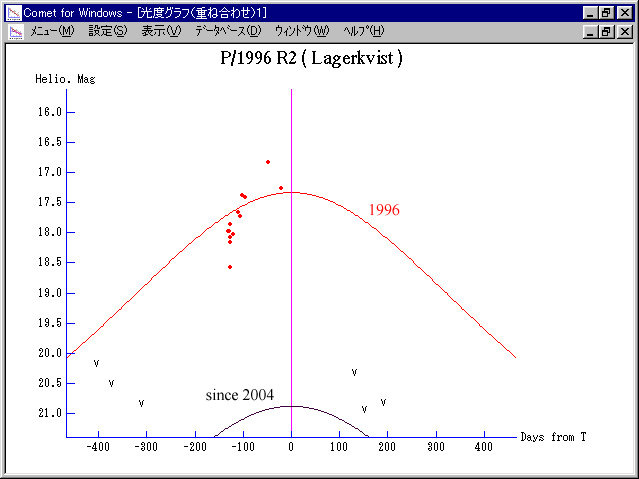###\$B2s5"5-O?(B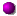\$BH/8+(B\$B=P8=(B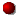\$B4QB,\$5\$l\$J\$+\$C\$?2s5"(B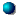\$BH/8+A0\$N=P8=(B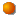\$BH/8+A0\$N4QB,\$5\$l\$J\$+\$C\$?2s5"(B\$B>-Mh\$N2s5"(B2019/02/112011/10/172004/06/07P/1996 R2 1997/01/19##### \$BH/8+;v>p\$HNr;K\$O(BGeneral Comet Info (Gary W. Kronk)\$B\$K\$"\$k!#(B \$BLZ2<0lCK;a\$N7W;;\$K\$h\$k2a5n\$HL\$Mh\$N50F;MWAG(B(http://www9.ocn.ne.jp/~comet/pcmt/j96r2.htm)\$B!#(B